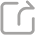分享至QQ空间 分享至朋友圈 分享至新浪微博

### Wave models with time-dependent coefficients

First we introduce different wave models with time-dependent coefficients. We discuss the behavior of suitable wave-type energies. Then we motivate models from cosmology with integrable in time speed of propagation. We introduce for a family of linear models a classification of damping terms and prove energy estimates. Finally, we discuss the optimality of the obtained estimates by applying the theory of special functions to scale-invariant models.